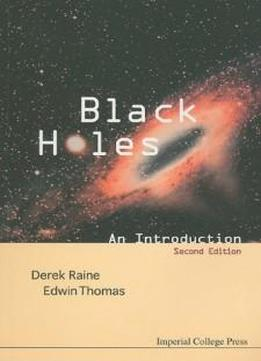# Black Holes: An Introduction by Derek Raine / 2009 / English / DjVu

This introduction to the fascinating subject of black holes fills a significant gap in the literature which exists between popular, non-mathematical expositions and advanced textbooks at the research level. It is designed for advanced undergraduates and first year postgraduates as a useful stepping-stone to the advanced literature. The book provides an accessible introduction to the exact solutions of Einstein's vacuum field equations describing spherical and axisymmetric (rotating) black holes. The geometry and physical properties of these spacetimes are explored through the motion of particles and light. The use of different coordinate systems, maximal extensions and Penrose diagrams is explained. The association of the surface area of a black hole with its entropy is discussed and it is shown that with the introduction of quantum mechanics black holes cease to be black and can radiate. This result allows black holes to satisfy the laws of thermodynamics and thus be consistent with the rest of physics. In this new edition the problems in each chapter have been revised and solutions are provided. The text has been expanded to include new material on wormholes and clarify various other issues.

This introduction to the fascinating subject of black holes fills a significant gap in the literature which exists between popular, non-mathematical expositions and advanced textbooks at the research level. It is designed for advanced undergraduates and first year postgraduates as a useful stepping-stone to the advanced literature. The book provides an accessible introduction to the exact solutions of Einstein's vacuum field equations describing spherical and axisymmetric (rotating) black holes. The geometry and physical properties of these spacetimes are explored through the motion of particles and light. The use of different coordinate systems, maximal extensions and Penrose diagrams is explained. The association of the surface area of a black hole with its entropy is discussed and it is shown that with the introduction of quantum mechanics black holes cease to be black and can radiate. This result allows black holes to satisfy the laws of thermodynamics and thus be consistent with the rest of physics. In this new edition the problems in each chapter have been revised and solutions are provided. The text has been expanded to include new material on wormholes and clarify various other issues.

views: 629